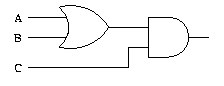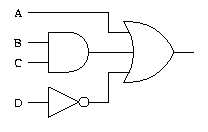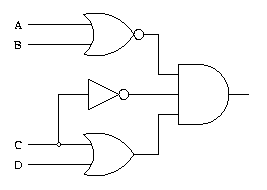## Wednesday, October 9, 2013

### Basic Logic Gates Problems

• Draw a logic circuit for (A + B)C.• Draw a logic circuit for A + BC + D.• Draw a logic circuit for AB + AC.• Draw a logic circuit for (A + B)(C + D)C.Source : http://sandbox.mc.edu